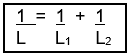# Inductors in Series and Parallel

### Inductors in Series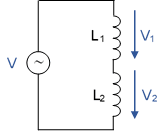Inductors in Series

We consider two coils connected in series, which are connected to AC voltage.

According to the rules of series connection:

V = V1  +  V2    Eq. (1)

We remember: Due to the law of self-induction, the voltage U caused on the coil (due to the change in time that we just have with AC voltage) can be written as follows: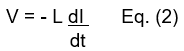Eq. (2) in Eq. (1) and you get: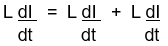Note: The minus sign has been shortened. According to the rules of series connection we have only "one" current. By further shortening it follows: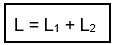### Inductors in ParallelInductors in Parallel

According to the rules of parallel connection applies:

I = I1 + I2                Eq. (3)

Since we are considering here the behavior of inductors at AC voltage, we form the differentiation of Eq. (4):

dI = dI1  +  dI2        Eq. (5)
dt    dt       dt

from V = - L dI       it follows    dI =  - V    Eq. (6)
dt                         dt        L

Eq. (6) in Eq. (5), the minus sign is shortened. You get:

V  =  V  +  V
L       L1     L2

According to the rules of parallel connection, we have only one voltage. Thus follows: